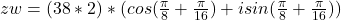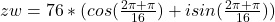Question

Let z =38 (cos(pi/8)+ i sin(pi/8)) and w = 2 (cos( pi/16 ) + i sin(pi/16))

What is the product of zw?

1.Philomena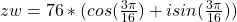Step-by-step explanation:

Given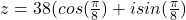and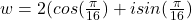Required

Determine zw

Given that: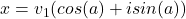and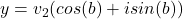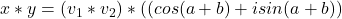So, we have: